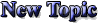Announcement: Be excellent to each other.

 Poster Message
Ezlo
Level: Smitemaster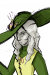Rank Points: 1192
Registered: 01-08-2006
IP: Logged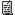Re: Stupid Math formulas (0)
I wouldn't go that far. You never know, maybe I just put up with your evilness because you scare all the annoying people away.
05-24-2006 at 06:56 PM
jbluestein
Level: Smitemaster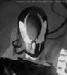Rank Points: 1644
Registered: 12-23-2005
IP: LoggedRe: Stupid Math formulas (0)
quote:
key-smiter wrote:
quote:
Pilchard VIII wrote:
I can prove that Barney the dinosaur is the devil!

Yes, and in the 1980s one could prove that Reagan was the Antichrist . . . his full name was Ronald Wilson Reagan. Each part of his name had six letters, ergo 666.

Regarding Reagan: the 666 letter count isn't hard to reach. What was more unfortunate in his case was the fact that Ronald Wilson Reagan is an anagram for Insane Anglo Warlord. (And no, this isn't political commentary -- please don't take it as such, or an invitation to such.)

Regarding Barney: my two-year-old son recently heard a friend of mine make the comment that Barney was evil. He promptly spoke up: "Don't say that! Barney is a nice boy!" Well, he's two. We don't much like Barney, but you can't really discuss issues of taste with toddlers.

____________________________
"Rings and knots of joy and grief, all interlaced and locking." --William Buck
05-24-2006 at 07:00 PM
Ravon
Level: Master Delver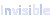Rank Points: 220
Registered: 02-19-2004
IP: LoggedRe: Stupid Math formulas (+1)
The first hand on a clock is the hour hand.
The second hand on a clock is the minute hand.
The third hand on a clock is the second hand.

so, second hand = minute hand
second = minute (you just divide by hand)
60 minutes = an hour
60 seconds = an hour (substitution)
60 seconds = a minute
minute = hour
second = minute = hour

The sky's the limit from there.
06-04-2006 at 07:26 PM
Someone Else
Level: Smitemaster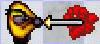Rank Points: 1177
Registered: 06-14-2005
IP: LoggedRe: Stupid Math formulas (0)
Are you saying time is imaginary?
Then it would be just like math, as I explained earlier.
06-05-2006 at 11:06 PM
key-smiter
Level: Master Delver
Rank Points: 121
Registered: 06-15-2003
IP: LoggedRe: Stupid Math formulas (+1)
This isn't stupid, per se, but timely -

Suppose you add up the squares of the first seven primes -

2^2 + 3^2 + 5^2 + 7^2 + 11^2 + 13^2 + 17^2

what do you get?
Click here to view the secret text

06-06-2006 at 04:29 PM
davis
Level: Roachling
Rank Points: 11
Registered: 12-13-2005
IP: LoggedRe: Stupid Math formulas (+1)
quote:
Kevin_P86 wrote:
There's another "proof" I have run across that "proves" 2 = 1:

a = b
a^2 = ab
a^2 - b^2 = ab - b^2
(a - b)(a + b) = b(a - b)
a + b = b
b + b = b
2b = b
2 = 1

------------------------------------------

Unfortunately this assumption is wrong because assuming that a=b, it is impossible to divide by (a-b) between
(a-b)(a+b)=b(a-b) and a+b=b
because a-b=0 and you cannot divide by zero.

____________________________
Ax+Bx=C
y=mx+b
06-06-2006 at 09:04 PM
DiMono
Level: Smitemaster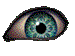Rank Points: 1161
Registered: 09-13-2003
IP: LoggedRe: Stupid Math formulas (+1)
Mathematical proof that studying is bad for you:
```        no study = fail
study = no fail
study + no study = fail + no fail
(1 + no) study = (1 + no) fail
study = fail```

____________________________
Deploy the... I think it's a yellow button... it's usually flashing... it makes the engines go... WHOOSH!
06-14-2006 at 11:47 AM
mrimer
Level: Legendary SmitemasterRank Points: 4458
Registered: 02-04-2003
IP: LoggedRe: Stupid Math formulas (0)
Substitute study for 1 and fail for 1? Hrmph.

____________________________
Gandalf? Yes... That's what they used to call me.
Gandalf the Grey. That was my name.
I am Gandalf the White.
And I come back to you now at the turn of the tide.
06-14-2006 at 03:04 PM
eytanz
Level: Smitemaster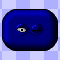Rank Points: 2707
Registered: 02-05-2003
IP: LoggedRe: Stupid Math formulas (+1)
quote:
mrimer wrote:
Substitute study for 1 and fail for 1? Hrmph.

I'm not sure what you mean by this, but there are two ways of interpreting DiMono's equation. One is absolutely correct, and the second is false because of a divide by 0 error.

The difference depends on the interpretation of "no". If "no" = 0 then you have:

0 x study = fail
study = 0 x fail
study + 0 x study = fail + 0 x fail
(1 + 0) x study = (1 + 0) x fail
study = fail

Since the first two formulae mean that both study and fail = 0, then this is prefectly ok.

If you interpret "no" = -, then you have:

-study = fail
study = -fail
study - study = fail - fail
(1-1) x study = (1-1) x fail
study = fail

But you can't simplify by (1-1), so the last line is invalid.

____________________________
I got my avatar back! Yay!
06-14-2006 at 04:31 PM
Someone Else
Level: SmitemasterRank Points: 1177
Registered: 06-14-2005
IP: LoggedRe: Stupid Math formulas (0)
quote:
davis wrote:
quote:
Kevin_P86 wrote:
There's another "proof" I have run across that "proves" 2 = 1:

a = b
a^2 = ab
a^2 - b^2 = ab - b^2
(a - b)(a + b) = b(a - b)
a + b = b
b + b = b
2b = b
2 = 1

------------------------------------------

Unfortunately this assumption is wrong because assuming that a=b, it is impossible to divide by (a-b) between
(a-b)(a+b)=b(a-b) and a+b=b
because a-b=0 and you cannot divide by zero.

But mine proves you can divide by zero.
06-15-2006 at 06:58 PM
keelan.m
Level: Roachling
Rank Points: 10
Registered: 06-03-2011
IP: LoggedRe: Stupid Math formulas (0)
quote:
KevG wrote:
quote:
Kevin_P86 wrote:A = B indeed does not imply sqrt(A) = sqrt(B)
Actually, A = B does imply sqrt(A) = sqrt(B). sqrt(A) is defined to be the positive root. This is a different statement from A^2 = B^2 does not imply A = B.

if a^2=b^2 then a obviously = b... and why is everyone called kevin...

____________________________
keelan

[Last edited by keelan.m at 06-03-2011 12:52 AM]
06-03-2011 at 12:52 AM
west.logan
Level: Smitemaster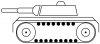Rank Points: 585
Registered: 03-09-2011
IP: LoggedRe: Stupid Math formulas (0)
Joking?

-1 != 1, but (-1)^2= 1^2...

____________________________
-Logan
06-03-2011 at 01:09 AM
Trickster
Level: SmitemasterRank Points: 658
Registered: 07-03-2007
IP: LoggedRe: Stupid Math formulas (+1)
quote:
Someone Else wrote:
anything*0=0 therefore
0/0=anything therefore
Argh! I can't believe I'm bumping this, but, no. You got it backwards.

(anything * 0 = 0) implies (0 / anything = 0).

In order for 0 / 0 = "anything" to be true, 0 * 0 = "anything" would have to be true, and it would have to be the only way to get "anything" by multiplying a number by 0 (which is why 0/0 =/= 0).

You can extend any commutative ring into a wheel (e.g. a set of numbers where division is always defined) by adding some extra numbers, but you lose some common algebraic properties if you do (division is no longer identical to multiplicative inverse, x - x is not always equal to 0, etc).

____________________________
Trickster

Official Hold Progress
Click here to view the secret text

Favorite Unofficial Holds (I need to play more!)
Click here to view the secret text

My Holds
Click here to view the secret text

03-01-2012 at 08:08 PM
stigant
Level: Smitemaster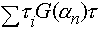Rank Points: 1173
Registered: 08-19-2004
IP: LoggedRe: Stupid Math formulas (0)
quote:
In order for 0 / 0 = "anything" to be true, 0 * 0 = "anything"

um, why? Wouldn't 0/0 = any imply that 0 * any = 0? (That's true, by the way)

Also, I don't think the intention here was to define "anything" as a new number (like adding infinity to the real numbers). Since division is defined as a/b = x iff a = b*x, and 0*x = 0 for all numbers, 0/0 = x would be true for all values of x. Since we want our operators to have only one output for any given pair of inputs, we decide that 0/0 is undefined. This is in contrast to x/0 (x =/= 0) which is undefined because there NO values of b such that b*0 = x (x =/= 0).

all of which is to say that the error in the proof at the beginning of this thread is mistaking the fact that "anything" refers to a set of numbers, not a particular number. It is true that anything (the set) = anything (the set), (since they both have the same members), but it's not true that a particular element of anything is equal to different member of anything.

____________________________
Progress Quest Progress

[Last edited by stigant at 03-01-2012 09:59 PM]
03-01-2012 at 09:32 PM
Someone Else
Level: SmitemasterRank Points: 1177
Registered: 06-14-2005
IP: LoggedRe: Stupid Math formulas (+1)
Actually, 0/0 is undefined because of this:
0/0 is equivalent to 0*(0^-1), because division is defined as multiplication by the inverse. Then (0^-1)=1/0, which is undefined because x does not exist for x*0=1.
03-01-2012 at 10:33 PM
Trickster
Level: SmitemasterRank Points: 658
Registered: 07-03-2007
IP: LoggedRe: Stupid Math formulas (0)
quote:
stigant wrote:
um, why? Wouldn't 0/0 = any imply that 0 * any = 0? (That's true, by the way)
I will assume that we're using real numbers with the usual meanings for *, /, and =. First off, the "=" predicate tests two real numbers for equality and evaluates to true or false.

By "any" you could mean, "There exists a real number 'any' such that (0 / 0 = any)", or you could mean, "For all real numbers 'any' it is true that (0 / 0 = any)". I'll explain why both of these propositions are false under the interpretation given above.

It's easiest to examine the second one first. The binary predicate "=" is a theorem of logic and without qualification refers to true equality. This means it must be reflexive (for all a, a = a), symmetric (a = b implies b = a), and transitive (if a = b and b = c, then a = c). Additionally, for it to be true equality rather than a mere equivalence relation, it must be the case that (a = b) implies for any equation f: f(x,a) = f(x,b).

So, by logic, and using a couple of axioms of natural numbers, I can show 0 =/= 1 on the natural numbers:

Axiom 1: for all x, (x + 1 =/= x)
Axiom 2: for all x, (x + 0 = x)
for all x, x + 1 =/= x + 0 (by transitivity of =)
0 + 1 =/= 0 + 0 (choose 0)
1 =/= 0 (apply axioms again)
0 =/= 1 (by symmetry of =)

Let's apply this to the latter of the two possibilities first.

"For all real numbers 'any' it is true that (0 / 0 = any)." If there exists more than one distinct real number in our universe, this must be false. Otherwise, you would have, for some x and some y =/= x:

0 / 0 = x
0 / 0 = y
x = y (by transitivity of =)

But this contradicts our assertion that there are two distinct real numbers x and y, so it must be false that 0 / 0 yields multiple solutions.

This shouldn't be surprising, however. Note that "/" is a binary function on real numbers. That means it must return a single real number anywhere it is defined. If it didn't return a single real number, you wouldn't be able to use "/" on two numbers to get a result that you could compare with "=".

Now let's consider the former statement:

"There exists a (particular) real number 'any' such that (0 / 0 = any)."

This one could be true by logic, and in fact it is possible to extend the reals to make it true (but rarely useful so it is not often done). However, it is not true under the typical interpretation of "/" as "the inverse function of *". Why is that? Simply put: there is no single number, that when multiplied by zero, returns zero. Multiplication on the reals is not an fully invertible function. If you want to treat "0 / 0" as a real number, it has to be a particular number. It can't be all of them, or you wouldn't be able to use it in calculations.

Essentially, +, -, *, and / are functions that take two specific terms and evaluate them to produce a third term. You can't treat (0/0) (or x/0 for any real number x) like a real number because there is no real number that maintains its algebraic properties and still satisfies what division by zero implies. You can't even define (0/0) to be 0 or -1 or 42 or some arbitrary value, because this will violate other algebraic properties and lead to immediate contradictions:

Assume: we can define (0/0) = a
For all x, 0 * x = 0 (axiom)
0 * 1 = 0 (substitute 1)
0 * 2 = 0 (substitute 2)
0 * 1 = 0 * 2 (substitute 0*2)
1 = 2 (divide by zero, since /0 is inverse of *0)

You might be tempted to say this contradiction only proves that (0/0) =/= 1, but if that's true then / is not the inverse of multiplication, so we're no longer talking about division at all.

Here's the bottom line. Math is the philosophical study of what happens logically when we decide to adopt certain rules. When those rules are inconsistent, they are worthless to us because once you produce an inconsistency you can prove anything from it, so all statements become true (0=1, 0=/=0, minimike is the best forum member ever). This works as follows, and is a basic tenet of logic:

1) For all x and y, "x implies y" is vacuously true whenever x is false. (If dragons exist, "blah". This statement is always true if dragons don't exist, because there can't be a contradiction unless "blah" is false and dragons exist. Basic logic, though a little different from how humans use "if" in English.)

2) Assume a contradiction (something that can be shown logically to be false) is proven true.

3) Therefore, every statement is true. (Just stick the contradiction in place of x, and what you want to say is true in place of y.)

Ironically, your proof that "math is absurd when we use 0/0" is a very good proof of why we choose to leave 0/0 undefined.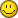Congrats!

____________________________
Trickster

Official Hold Progress
Click here to view the secret text

Favorite Unofficial Holds (I need to play more!)
Click here to view the secret text

My Holds
Click here to view the secret text

[Last edited by Trickster at 03-01-2012 10:38 PM]
03-01-2012 at 10:37 PM